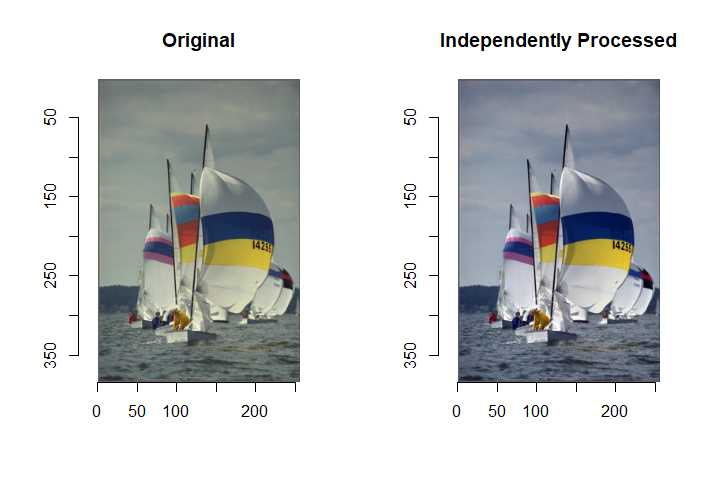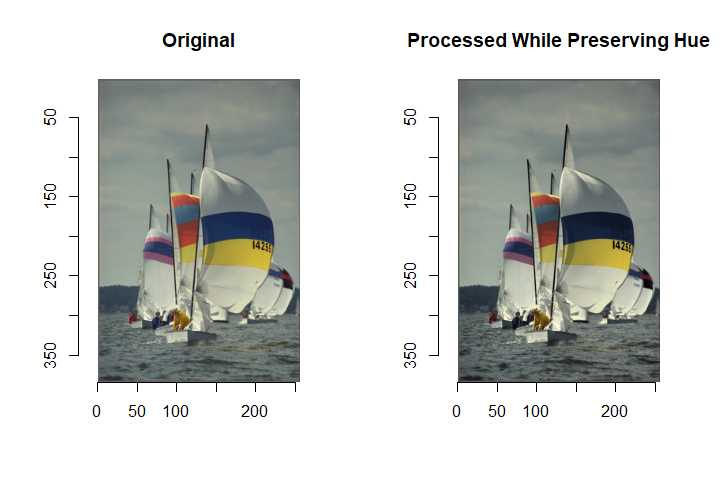Treating Color Image with imagerExtra

2019-01-25

We have two options when treating color images with imagerExtra.

• process the channels independently
• preserve the hue of image, process the intensity component and then compute RGB values from the new intensity component

The former is straightforward.

One example is shown below.

library(imagerExtra)
x <- boats
s <- 0.1
R(x) <- BalanceSimplest(R(x), s, s, range=c(0,1))
G(x) <- BalanceSimplest(G(x), s, s, range=c(0,1))
B(x) <- BalanceSimplest(B(x), s, s, range=c(0,1))
layout(matrix(1:2, 1, 2))
plot(boats, main = "Original")
plot(x, main = "Independently Processed")The latter needs three functions: Grayscale, GetHue, RestoreHue.

• Grayscale: computes average of RGB channel
• GetHue: stores hue of image
• RestoreHue: restores hue of image

grayscale function of imager computes as shown below by default.

Y = 0.300000R + 0.590000G + 0.110000B

where Y is grayscale value, R is R value, G is G value, and B is B value.

This equation reflects the way of human visual perception.

This grayscale conversion makes it difficult to restore hue of image.

That’s why we need Grayscale function, which just compute average of RGB channels.

How to use these functions is shown below.

g <- Grayscale(boats)
hueim <- GetHue(boats)
g <- BalanceSimplest(g, s, s, range=c(0,1))
y <- RestoreHue(g, hueim)
layout(matrix(1:2, 1, 2))
plot(boats, main = "Original")
plot(y, main = "Processed While Preserving Hue")Which way is better?